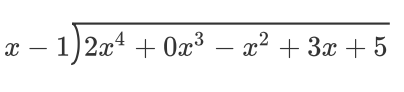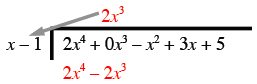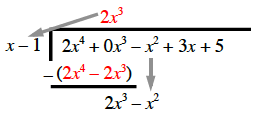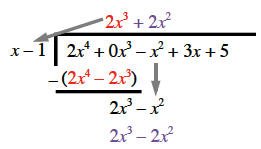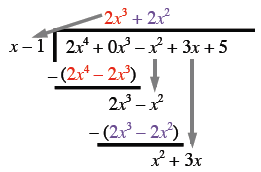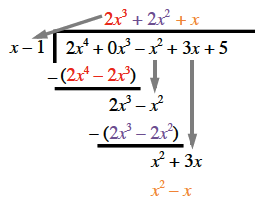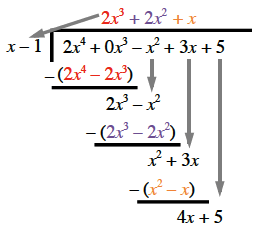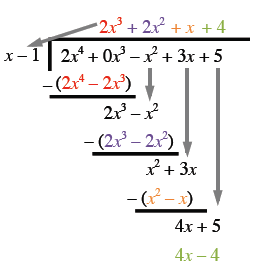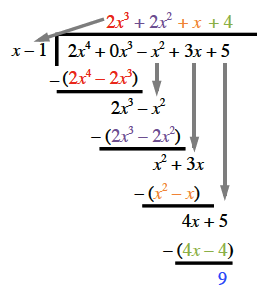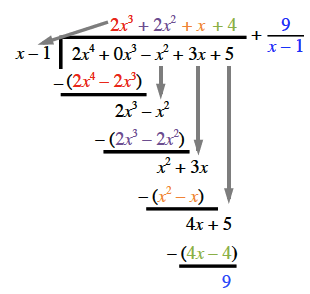### Home > PC3 > Chapter 5 > Lesson 5.1.2 > Problem5-23

5-23.

Complete the following division problem. Express any remainder as a fraction.

$\frac { 2 x ^ { 4 } - x ^ { 2 } + 3 x + 5 } { x - 1 }$

Set up the problem. Long Division format, side labeled, x minus 1, Inside labeled, 2 x to the fourth, + 0 x cubed, minus x squared, + 3 x, + 5

Determine the first term of the quotient and multiply. Added above second term, in red is, 2 x cubed, with arrow pointing to left side, & second line, under first & second terms, in red is, 2 x to the fourth, minus 2 x cubed.

Subtract and bring the next term down. Added, parentheses around 2 x to the fourth minus 2 x cubed, with negative sign in front of left parenthesis, & underscore below, aligned under second & third terms, is 2 x cubed minus x squared, with down arrow aligning third terms, from top line to bottom line.

Determine the next term of the quotient and multiply. Added, above third term, purple 2 x squared, & fourth line, aligned below second & third terms, purple 2 x cubed, minus 2 x squared.

Subtract and bring the next term down. Added, parentheses around purple fourth line, with negative sign in front, & underscore below, fifth line, aligned under third & fourth, x squared, + 3 x, with down arrow aligning fourth terms, from top to bottom lines.

Determine the next term of the quotient and multiply. Added, above fourth term, orange, + x, & sixth line, aligned below third & fourth terms, orange x squared, minus x.

Subtract and bring the next term down. Added, parentheses around orange sixth line, with negative sign in front, & underscore below, seventh line under fourth & fifth terms, 4 x, + 5, with down arrow aligning fifth terms, from top to bottom lines.

Determine the next term of the quotient and multiply. Added above fifth term, green + 4, & eighth line, aligned below fourth & fifth terms, green, 4 x, minus 4.

Subtract. Added, parentheses around green eighth line, with negative in front, & underscore below, ninth line under fifth terms, blue 9.

Write the remainder as a fraction. Added, top line, in blue, + 9 divided by quantity x minus 1.